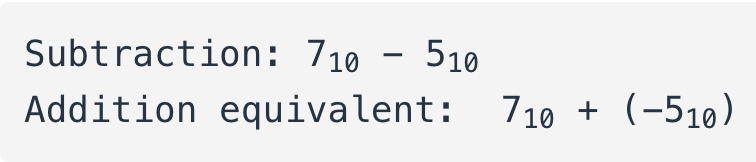## How do you subtract one binary number from another?

Using the standard techniques adapted for decimal numbers (subtraction of each bit pair, right to left, “borrowing” as needed from bits to the left)

# Binary Subtraction

## Chapter 2 - Binary Arithmetic

We can subtract one binary number from another by using the standard techniques adapted for decimal numbers (subtraction of each bit pair, right to left, “borrowing” as needed from bits to the left). However, if we can leverage the already familiar (and easier) technique of binary addition to subtract, that would be better.

As we just learned, we can represent negative binary numbers by using the “two’s complement” method and a negative place-weight bit. Here, we’ll use those negative binary numbers to subtract through addition.

Here’s a sample problem:

Subtraction: 710 - 510


If all we need to do is represent seven and negative five in binary (two’s complement) form, all we need is three bits plus the negative-weight bit:

positive seven = 01112
negative five  = 10112


.                    1111  <--- Carry bits
.                     0111
.                   + 1011
.                   ------
.                    10010
.                    |
.


Since we’ve already defined our number bit field as three bits plus the negative-weight bit, the fifth bit in the answer (1) will be discarded to give us a result of 00102, or positive two, which is the correct answer.

Another way to understand why we discard that extra bit is to remember that the leftmost bit of the lower number possesses a negative weight, in this case equal to negative eight.

When we add these two binary numbers together, what we’re actually doing with the MSBs is subtracting the lower number’s MSB from the upper number’s MSB. In subtraction, one never “carries” a digit or bit on to the next left place-weight.

Let’s try another example, this time with larger numbers. If we want to add -2510 to 1810, we must first decide how large our binary bit field must be.

To represent the largest (absolute value) number in our problem, which is twenty-five, we need at least five bits, plus a sixth bit for the negative-weight bit. Let’s start by representing positive twenty-five, then finding the two’s complement and putting it all together into one numeration:

+2510  = 0110012 (showing all six bits)
One's complement of 110012 = 1001102
One's complement + 1 = two's complement = 1001112
-2510 = 1001112


Essentially, we’re representing negative twenty-five by using the negative-weight (sixth) bit with a value of negative thirty-two, plus positive seven (binary 1112).

.              1810  = 0100102
.
.       Now, let's add them together and see what we get:
.
.                     11   <--- Carry bits
.                   100111
.                 + 010010
.                 --------
.                   111001


Since there were no “extra” bits on the left, there are no bits to discard. The leftmost bit on the answer is a 1, which means that the answer is negative, in two’s complement form, as it should be. Converting the answer to decimal form by summing all the bits times their respective weight values, we get:

(1 x -3210)  +  (1 x 1610)  +  (1 x 810)  +  (1 x 110)  = -710


Indeed -710 is the proper sum of -2510 and 1810.

RELATED WORKSHEETS:

• Share
Published under the terms and conditions of the Design Science License